Courses

# Fluid Machinery - 1

## 20 Questions MCQ Test Mock Test Series for SSC JE Mechanical Engineering | Fluid Machinery - 1

Description
This mock test of Fluid Machinery - 1 for SSC helps you for every SSC entrance exam. This contains 20 Multiple Choice Questions for SSC Fluid Machinery - 1 (mcq) to study with solutions a complete question bank. The solved questions answers in this Fluid Machinery - 1 quiz give you a good mix of easy questions and tough questions. SSC students definitely take this Fluid Machinery - 1 exercise for a better result in the exam. You can find other Fluid Machinery - 1 extra questions, long questions & short questions for SSC on EduRev as well by searching above.
QUESTION: 1

### Equation of continuity results from the principal of conservation of _____.

Solution:

The law of conservation of mass states that mass can neither be created nor be destroyed.

Rate at which mass enters the region = Rate at which mass leaves the region + Rate of accumulation of mass in the region

The above statement can be expressed analytically in terms of velocity and density field of a flow and the resulting expression is known as the equation of continuity or the continuity equation.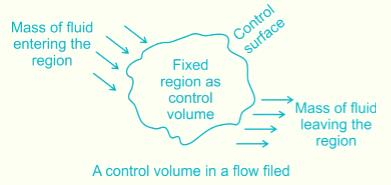$$\begin{array}{l} \frac{{\partial \rho }}{{\partial t}} + \frac{\partial }{{\partial x}}\left( {\rho u} \right) + \frac{\partial }{{\partial y}}\left( {\rho v} \right) + \frac{\partial }{{\partial z}}\left( {\rho w} \right) = 0\\ \frac{{\partial \rho }}{{\partial t}} + .\left( {{\rm{\rho \vec V}}} \right) = 0 \end{array}$$

QUESTION: 2

### Which of the following conditions will be satisfied by steady irrotational flow?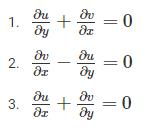Solution:

For steady, irrotational flow following two condition must be fulfilled:

i) flow possibility

ii) ωz = 0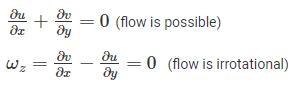QUESTION: 3

### An object weighs 60 gm in air, 50 gm in water and 40 gm in oil. Then the specific gravity of the oil will be _____.

Solution:

Weight of solid in air=60gm

Weight of solid in water=50gm

Weight of solid in oil=40gm

Specific gravity=Relative density of liquid=?

Relative density of liquid
=loss of weight of solid in liquid/loss of weight of solid in water

=(60-40)/50

=20/50

=0.4

QUESTION: 4

The shear stress-strain graph for a Newtonian fluid is a

Solution:

Newtonian fluids defined as fluids for which the shear stress is linearly proportional to the shear strain rate. Newtonian fluids are analogous to elastic solids (Hooke’s law: stress proportional to strain). Any common fluids, such as air and other gases, water, kerosene, gasoline, and other oil-based liquids, are Newtonian fluids.

Fluids for which the shear stress is not linearly related to the shear strain rate are called non- Newtonian fluids. examples include slurries and colloidal suspensions, polymer solutions, blood, paste, and cake batter.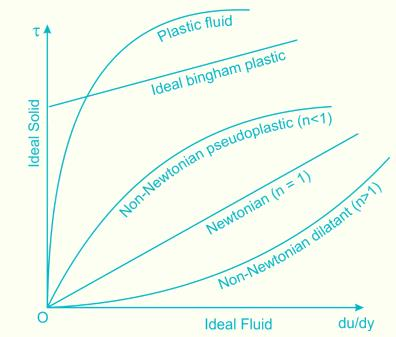QUESTION: 5

The mechanical efficiency of an impulse turbine is generally between:

Solution:

Mechanical efficiency of Impulse turbine is given by: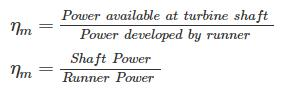It generally varies between 97 to 99

Also, volumetric efficiency (ηv) = 97 to 99

Overall efficiency (ηo) = 85 to 90

QUESTION: 6

An increase in pressure of a liquid from 7.5 MPa to 15 MPa results into 0.2 percent decrease in its volume. The coefficient of compressibility of the liquid in m2/N is

Solution:

dV = 0.2%

Compressibility (β) =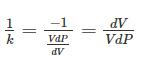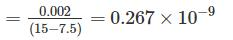QUESTION: 7

Which one of the following statement is CORRECT about the centre of buoyancy?

Solution:

Objects in a fluid experience an upward force. Whenever an object is immersed in a fluid, either liquid or gas, it experiences a buoyant force. A buoyant force is a force which pushes upward on an object and is caused by displaced fluid.

The centre of buoyancy is the point where the resultant Buoyant force acts, It is the point of the C.G of the displaced fluid.

QUESTION: 8

Property of a fluid by which molecules of different kinds of fluids are attracted to each other is called _____.

Solution:
• Adhesion is the mutual attraction between unlike molecules that causes them to cling to one another
• Capillary action and meniscus (the curved surface which is formed by any liquid in a cylinder) are the effects of adhesion
• Cohesion is the mutual attraction between like molecules that causes them to stick together.
• Surface tension, Capillary action, and meniscus are the effects of cohesion.
QUESTION: 9

In which type of the following flow, loses are more?

Solution:

Losses are governed by hf

For laminar flow, hf α f2

Where f1 = friction factor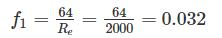Put Re = 2000

Forturbulentflow,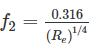Even for Re = 2000

f2 = 0.039

f2 > f1

So, losses are more in turbulent flow.

QUESTION: 10

While representing the energy equation, the difference between the total head line and Hydaullic gradient line is:

Solution:

The difference between the total head line and Hydraullic gradient line is always constant for particular pipe flow and it is equal to the velocity head.

QUESTION: 11

Which one of the following equations represents the continuity equation for steady compressible fluid flow?

Solution:

for steady flow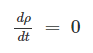Continuity equation for steady compressible flow is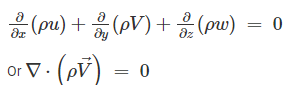QUESTION: 12

What is the value of the angle (degree) between stream lines and equipotential lines at the point of intersection in the flow net?

Solution:

Angle between stream lines and equipotential lines at a point of intersection in the flow net is always intersect at 90°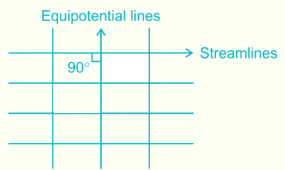QUESTION: 13

An oil of kinematic viscosity of 0.5 stokes flow through a pipe of 20 cm diameter. What is the velocity if the flow is critical?

Solution:

ν = 0.5 × 10-4 m2/s

D = 20 cm

For critical,  Re = 2000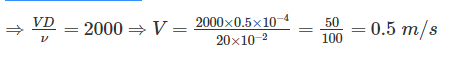QUESTION: 14

For very high discharge at low pressure such as for flood control and irrigation applications, which of the following types of pump is preferred?

Solution:

The main advantage of an Axial flow pump is that it has a relatively high discharge (flow rate) at a relative low head.

For example, it can pump up to 3 times more water and other fluids at lifts of less than 4 meters as compared to the more common radial - flow or centrifugal pump. They are used by smaller farmers for crop irrigation, drainage and fisheries.

QUESTION: 15

Calculate the diameter of a pipe of 32000 m long, if it is equivalent to another pipe of 0.2 m diameter and 1000 m long.

Solution:

Using dupit’s equation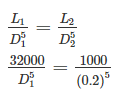D1 = 0.4 m

QUESTION: 16

The upper surface of a weir over which water flows is known as

Solution:

A weir may be defined as a structure constructed across a river or canal to store water on the upstream side. Water flows over the crest of weir.

The sheet of water flowing through a notch or over a weir is known as nappe or vein. The bottom edge of the notch or the top of a weir over which water flows is known as sill or crest. The height above the bottom of the tank or channel is known as crest height.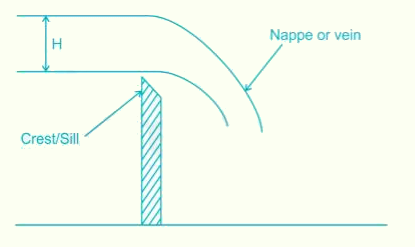QUESTION: 17

The fluid forces considered in the Navier-Strokes equation are ______.

Solution:

Navier-Strokes equation describe the motion of viscous fluid substances. These balance equations arise from applying Newton's second law to fluid motion, together with the assumption that the stress in the fluid is the sum of a diffusing viscous term (proportional to the gradient of velocity) and a pressure term-hence describing viscous flow.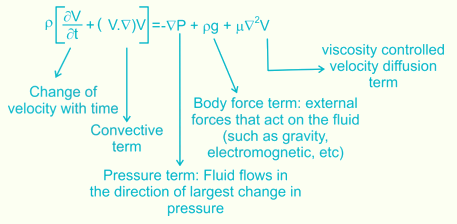QUESTION: 18

The standard sea level atmospheric pressure is equivalent to

Solution:

Pressure at sea level = 101.325 kPa

Checking according to given options.

P = hρg

a) ⇒ 10.2 m of fresh water (ρ = 998 kg/m3)

P = 10.2 × 998 × 9.81 = 99861.87 Pa = 99.861 kPa

b) ⇒ 10.1 m of saline water (ρ = 1025 kg/m3)

P = 10.1 × 1025 × 9.81 = 101.558 kPa

c) ⇒ 12.5 m of kerosene of ρ = 800 kg/m3

P = 12.5 × 800 × 9.81 = 98.1 kPa

d) ⇒ 6.4 m of carbon tetrachloride of ρ = 1590 kg/m3

⇒ 6.4 × 1590 × 9.81 = 99.826 kPa

So option b is nearest, so correct

QUESTION: 19

Which of the following instrument is used for measuring the discharge?

Solution:

Venturimeter: Used to measure discharge in the pipe flow.

Manometer: Measure low, medium and high gauge as well as vacuum pressure of liquids and gases both.

Current meter: Used to measure the velocity of water in the rivers

Vane anemometer: Used for measuring the velocity and volumetric flow rate on air grills in residential buildings and utility.

QUESTION: 20

For measuring flow by a Venturimeter, it should be installed in _______.

Solution:

Venturimeter is a device which measure flow rate of fluid through pipe. It can be used at any position.

When a venture meter is placed in a pipe carrying the fluid whose flow rate is to be measured, a pressure drop occurs between the entrance and throat of the venturimeter. This pressure drop is measured using a differential pressure sensor and when calibrated this pressure drop becomes a measure of flow rate.